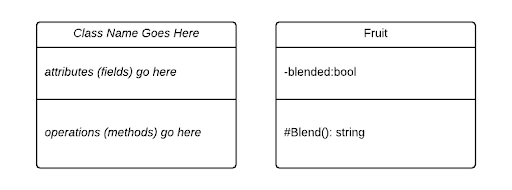# Classes

In a UML class diagram, individual classes are represented with a box divided into three compartments, each of which is for displaying specific information:The first compartment identifies the class - it contains the name of the class. The second compartment holds the attributes of the class (in C#, these are the fields and properties). And the third compartment holds the operations of the class (in C#, these are the methods).

In the diagram above, we can see the Fruit class modeled on the right side.

### Attributes

The attributes in UML represent the state of an object. For C#, this would correspond to the fields and properties of the class.

We indicate fields with a typed element, i.e. in the example above, the blended field is represented with:

$$\texttt{-blended:bool}$$

Indicating it should be declared private with the type bool.

For properties, we add a stereotype containing either get, set, or both. I.e. if we were to expose the private field bool with a public accessor, we would add a line to our class diagram with:

$$\texttt{+Blended:bool<<get,set>>}$$

### Operators

The operators in UML represent the behavior of the object, i.e. the methods we can invoke upon it. These are declared using the pattern:

$$\texttt{visibility name([parameter list])[:return type]}$$

The $\texttt{[visibility]}$ uses the same symbols as typed elements, with the same correspondences. The $\texttt{name}$ is the name of the method, and the $\texttt{[parameter list]}$ is a comma-separated list of typed elements, corresponding to the parameters. The $\texttt{[:return type]}$ indicates the return type for the method (it can be omitted for void).

Thus, in the example above, the protected method Blend has no parameters and returns a string. Similarly, the method:

public int Add(int a, int b)
{
return a + b;
}


Would be expressed:

$$\texttt{+Add(a:int, b:int):int}$$

## Static and Abstract

In UML, we indicate a class is static by underlining its name in the first compartment of the class diagram. We can similarly indicate static operators and methods is static by underlining the entire line referring to them.

To indicate a class is abstract, we italicize its name. Abstract methods are also indicated by italicizing the entire line referring to them.